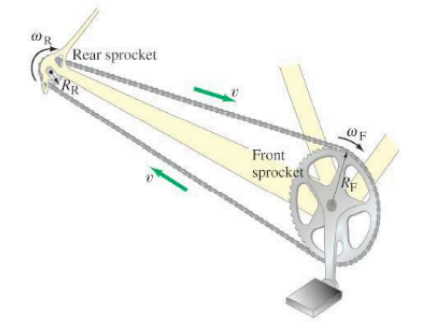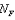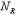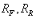×
Get Full Access to Physics: Principles With Applications - 6 Edition - Chapter 8 - Problem 73gp
Get Full Access to Physics: Principles With Applications - 6 Edition - Chapter 8 - Problem 73gp

×

# Bicycle gears: (a) How is the angular velocity wR of theISBN: 9780130606204 3

## Solution for problem 73GP Chapter 8

Physics: Principles with Applications | 6th Edition

• Textbook Solutions
• 2901 Step-by-step solutions solved by professors and subject experts
• Get 24/7 help from StudySoup virtual teaching assistantsPhysics: Principles with Applications | 6th Edition

4 5 1 262 Reviews
27
3
Problem 73GP

(a) For a bicycle, how is the angular speed of the rear wheel $$\left(\omega_{R}\right)$$ related to that of the pedals and front sprocket $$\left(\omega_{F}\right)$$, Fig.That is, derive a formula for $$\omega_{R} / \omega_{F}$$. Let $$N_{F} \text { and } N_{R}$$ be the number of teeth on the front and rear sprockets, respectively. The teeth are spaced equally on all sprockets so that the chain meshes properly.

(b) Evaluate the ratio $$\omega_{R} / \omega_{F}$$ when the front and rear sprockets have 52 and 13 teeth, respectively, andwhen they have 42 and 28 teeth.FIGURE 8-52 Problem 73.

Equation Transcription:Text Transcription:

(\omega_R)

(\omega_F)

\omega_R / \omega_F

NF and NR

\omega_R / \omega_F

Step-by-Step Solution:

Step 1 of 4

(A) Let the spacing between the teeth on the front and rear wheel be d, and the number of teeth on the front and the rear wheel areandrespectively.

Radii of the front and rear sprockets are.

Hence, we haveStep 2 of 4

Step 3 of 4

##### ISBN: 9780130606204

The answer to “?(a) For a bicycle, how is the angular speed of the rear wheel $$\left(\omega_{R}\right)$$ related to that of the pedals and front sprocket $$\left(\omega_{F}\right)$$, Fig. That is, derive a formula for $$\omega_{R} / \omega_{F}$$. Let $$N_{F} \text { and } N_{R}$$ be the number of teeth on the front and rear sprockets, respectively. The teeth are spaced equally on all sprockets so that the chain meshes properly. (b) Evaluate the ratio $$\omega_{R} / \omega_{F}$$ when the front and rear sprockets have 52 and 13 teeth, respectively, and when they have 42 and 28 teeth. FIGURE 8-52 73.Equation Transcription: Text Transcription:(\omega_R) (\omega_F) \omega_R / \omega_F NF and NR\omega_R / \omega_F” is broken down into a number of easy to follow steps, and 109 words. Since the solution to 73GP from 8 chapter was answered, more than 428 students have viewed the full step-by-step answer. This textbook survival guide was created for the textbook: Physics: Principles with Applications, edition: 6. The full step-by-step solution to problem: 73GP from chapter: 8 was answered by , our top Physics solution expert on 03/03/17, 03:53PM. Physics: Principles with Applications was written by and is associated to the ISBN: 9780130606204. This full solution covers the following key subjects: rear, teeth, front, sprocket, sprockets. This expansive textbook survival guide covers 35 chapters, and 3914 solutions.

## Discover and learn what students are asking

Calculus: Early Transcendental Functions : Inverse Trigonometric Functions: Integration
?In Exercises 1-20, find the indefinite integral. $$\int \frac{1}{x \sqrt{4 x^{2}-1}} d x$$

Statistics: Informed Decisions Using Data : Testing the Significance of the Least-Squares Regression Model
?The U.S. Population The following data represent the population of the United States for the years 1900–2010 An ecologist i

Unlock Textbook Solution